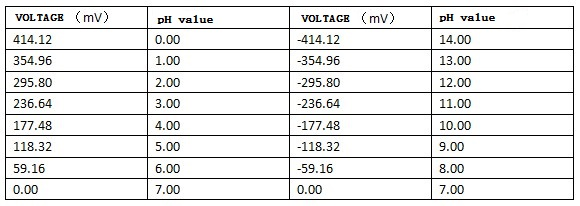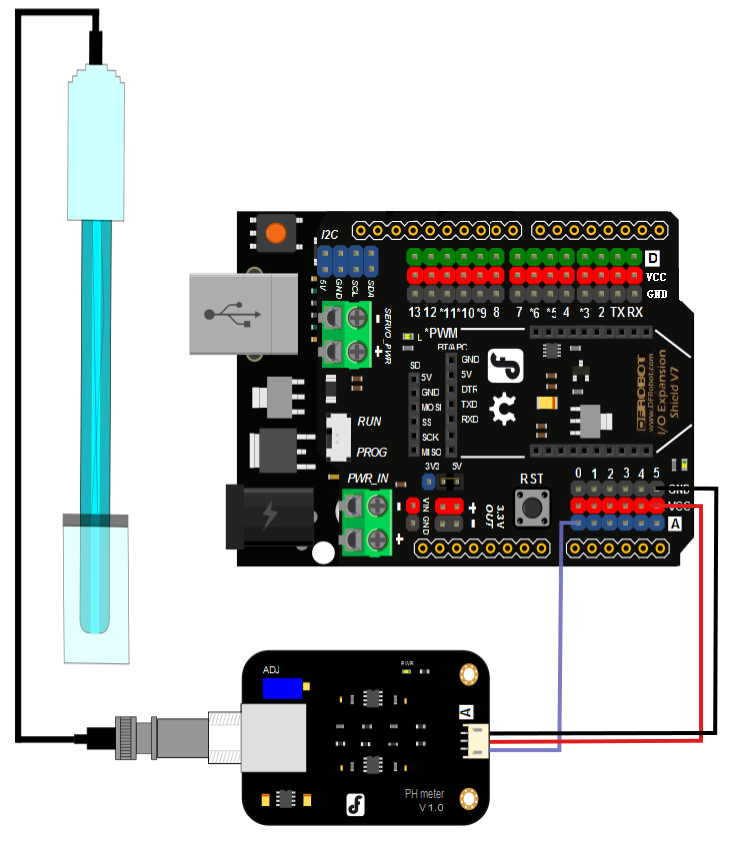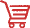# Analog_pH_Meter_Pro_SKU_SEN0169-DFRobot## Introduction

Here comes an analog pH meter Pro,specially designed for Arduino controllers. It use an industrial electrode and has a built-in simple, convenient, practical connection and long life, and overall is very suitable for online monitoring. It has an LED which works as the Power Indicator, a BNC connector and PH2.0 sensor interface. To use it, just connect the pH sensor with BND connector, then plug the PH2.0 interface into the analog input port of any Arduino controller. If programmed correctly, you will get the pH value easily. This industry standard pH combination electrode is made of sensitive glass membrane with low impedance. It can be used in a variety of PH measurements with fast response and good thermal stability. It has the good reproducibility, difficult to hydrolysis, and basically eliminate the alkali error. In 0 to 14pH range, the output voltage of the electrode is linear. The reference system which consist of the Ag/AgCl gel electrolyte salt bridge has a stable half-cell potential and excellent anti-pollution performance. The ring PTFE membrane is not easy to be clogged, so the electrode is suitable for long-term online detection. This pH meter pro is very suitable for long-term monitoring.

Attention: In order to ensure the accuracy of the pH probe, you need to use the standard solution to calibrate it regularly. Generally, the period is about half a year. If you meaure the dirty aqueous solution, you need to increase the frequency of calibration.

## Specification

• Module Power: 5.00V
• Module Size: 43mmx32mm
• Measuring Range: 0-14PH
• Measuring Temperature: 0-60 ℃
• Accuracy: ± 0.1pH (25 ℃)
• Response Time: ≤ 1min
• Industry pH Electrode with BNC Connector
• PH2.0 Interface ( 3 foot patch )
• Power Indicator LED

### pH Electrode Characteristics

The output of pH electrode is in millivolts, and the pH value of the relationship is shown as follows (25 ℃):## Use the pH Meter

### Connecting Diagram### Step to Use the pH Meter

Cautions:

• Please use an external switching power supply,and keep the voltage as close as possible to the +5.00V. The more accurate the voltage, the more accruate your reading is!

• Before placing the electrode in continuous use every time,you need to calibrate it by the standard solution to ensure accurate results.The best environment temperature is about 25 ℃,and the pH value is known and reliable and is close to the measured value. If you measure the acidic sample, the pH value of the standard solution should be 4.00.If you measure the alkaline sample, the pH value of the standard solution should be 9.18.Please look at the calibration subsection in order to get a better accuracy.

• Before the pH electrode can be used in different solutions, you need to use water to wash it. We recommend using deionized water.

1. Connect the equipment according to the above graphic, ensuring the pH electrode is connected to the BNC connector on the pH meter board，and the pH meter board is connected to ananlog port 0 on your Arduino controller. When the Arduino controller gets power,you will see the blue LED on the board is on.

2. Upload the sample code to the Arduino controller.

3. Put the pH electrode into the standard solution whose pH value is 7.00，or directl short the input of the BNC connector. Open the serial monitor of the Arduino IDE, and then you can see the pH value printed on it. Check to ensure the error does not exceed 0.3. Record the pH value printed,then compare that with 7.00. The difference should be changed in the "Offset" variable in the sample code. For example,the pH value printed is 6.88,so the difference is 0.12.You should change the "# define Offset 0.00" into "# define Offset 0.12" in your program.

4. Put the pH electrode into the pH standard solution whose value is 4.00. Then wait about one minute,adjust the gain potential device, let the value stabilise at around 4.00.At this time,the acidic calibration has been completed and you can measure the pH value of an acidic solution.

Note:If you want to measure the pH value of other solution,you must wash the pH electrode first!

1. According to the linear characteristics of pH electrode itself, after the above calibration,you can directly measure the pH value of the alkaline solution, but if you want to get better accuracy, you can recalibrate it. Alkaline calibration use the standard solution whose pH value is 9.18.Also adjust the gain potential device, let the value stabilise at around 9.18. After this calibration, you can measure the pH value of the alkaline solution.

### Sample Code

Sample code for testing the PH meter and get the sensor feedback from the Arduino Serial Monitor.

``````/*
# This sample code is used to test the pH meter Pro V1.0.
# Editor : YouYou
# Ver    : 1.0
# Product: analog pH meter Pro
# SKU    : SEN0169
*/
#define SensorPin A0            //pH meter Analog output to Arduino Analog Input 0
#define Offset 0.00            //deviation compensate
#define LED 13
#define samplingInterval 20
#define printInterval 800
#define ArrayLenth  40    //times of collection
int pHArray[ArrayLenth];   //Store the average value of the sensor feedback
int pHArrayIndex=0;
void setup(void)
{
pinMode(LED,OUTPUT);
Serial.begin(9600);
Serial.println("pH meter experiment!");    //Test the serial monitor
}
void loop(void)
{
static unsigned long samplingTime = millis();
static unsigned long printTime = millis();
static float pHValue,voltage;
if(millis()-samplingTime > samplingInterval)
{
if(pHArrayIndex==ArrayLenth)pHArrayIndex=0;
voltage = avergearray(pHArray, ArrayLenth)*5.0/1024;
pHValue = 3.5*voltage+Offset;
samplingTime=millis();
}
if(millis() - printTime > printInterval)   //Every 800 milliseconds, print a numerical, convert the state of the LED indicator
{
Serial.print("Voltage:");
Serial.print(voltage,2);
Serial.print("    pH value: ");
Serial.println(pHValue,2);
printTime=millis();
}
}
double avergearray(int* arr, int number){
int i;
int max,min;
double avg;
long amount=0;
if(number<=0){
Serial.println("Error number for the array to avraging!/n");
return 0;
}
if(number<5){   //less than 5, calculated directly statistics
for(i=0;i<number;i++){
amount+=arr[i];
}
avg = amount/number;
return avg;
}else{
if(arr<arr){
min = arr;max=arr;
}
else{
min=arr;max=arr;
}
for(i=2;i<number;i++){
if(arr[i]<min){
amount+=min;        //arr<min
min=arr[i];
}else {
if(arr[i]>max){
amount+=max;    //arr>max
max=arr[i];
}else{
amount+=arr[i]; //min<=arr<=max
}
}//if
}//for
avg = (double)amount/(number-2);
}//if
return avg;
}``````

### Precautions

• If the electrode is used for the first or after a long set without re-use, the electrode bulb and the sand core should be immersed in the 3NKCL solution for eight hours.

• The electrode plug should be kept clean and dry.

• Electrode reference solution is the 3NKCL solution.

• Measurement's of staggered pollution levels should be avoided, so as not to affect the accuracy of measurement.

• Electrode blub or sand core is defiled which will make PTS decline, and have a slow response. So, it should be based on the characteristics of the pollutant, then adapted to the cleaning solution to ensure electrode performance recovery.

• The electrode should not be long-term immersed in acid chloride solution.

• Electrode when in use, the ceramic sand core and liquid outlet rubber ring should be removed, in order to make salt bridge solution to maintain a certain velocity.

## More DocumentsGet Gravity: Analog pH Sensor / Meter Pro Kit For Arduino from DFRobot Store or DFRobot Distributor.

Turn to the Top